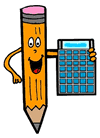# MATH RESOURCES

### ... from the Science Page

 A+ Math - offers java based games in addition, subtraction, division and multlipication. Ask Dr. Math - offers free frequently asked math questions and answers, math formulas, and sells math books. Calculators - provides a scientific calculator and a variety of math and health calculation tools. Circles - Math2 offers equasions and definitions for terms related to a circle. Geometry Junkyard - link list of a wide variety of Geometry resources. Introduction to Algebra - free content article with example problems explaining how algebra works.Learn to be a Human Calculator - free resources offering tips and advice on how to become a math whiz. Math Baseball - fun game used to help teach students addition, subtraction, division and multiplication. Math Calculators - various math related calculators from Math.com MathDork - offers free and for sale animated Algebra lessons. Math Games - a wide array of free online math games. Math Glossary - definitions of math terms organized alphabetically for each grade. MathWay - online math problem solver for basic math, algebra, geometry, trigonometry, calculus, statistics and finite math. Newton's Method - perl based script offering online answers to algebra problems. Nuts about Geometry - free Geometry glossary and activities ScienceU Geometry - interactive activities and information about Geometry. The Complete History of Calculators for Kids - guide explaining the history of calculation along with showing people how we use math and specialized calculators in everyday life. Wolfram Mathematica - the world's definitive system for modern technical computing Top of this page ..... or ..... Return to the SCIENCE PAGEE-mail me with suggestions for new sites or to indicate links that have changed. © 2001 - 2021